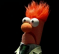Started by 5 years ago9 replieslatest reply 5 years ago223 views

The LTC2500-32 ADC has a so-called spread-sinc (ssinc) filter (datasheet). When googling the only references which turn up are from Linear it self. As far as I can tell it is a variant of a normal sinc filter. Does anyone know more about this filter, and how it was designed?

Kind regards,
Vincent

[ - ]Hello all,

I looked at the spectrum of one of the s-sinc filters: Once you see it, you know how the filter was designed. They are cascades of multiple CIC filters. instead of defining the filter as [(1-Z^(-M))/(1-Z^(-1))]^k we use one filter that appears to be zero packed to form multiple copies of the sinc spectral peak and then a second filter to drive down the multiple peaks:

the filter will look like

[(1-Z^(-4 M_1))/(1-Z^(-4))]^k_1 times [(1-Z^(-4))/(1-Z^(-1))]^k_2

run the following code to demonstrate above equation for M_1=6, k_1=8, k_2=4

fred harris

b1=[1 zeros(1,23) -1]; b2=conv(b1,b1); b3=conv(b2,b2);
bb=conv(b3,b3);
a1=[1 zeros(1,3) -1];  a2=conv(a1,a1); a3=conv(a2,a2);
aa=conv(a3,a3);
h1=filter(bb,aa,[1 zeros(1,160)]);

bb1=[1 zeros(1,3) -1]; bb2=conv(bb1,bb1);
bb3=conv(bb2,bb2);
aa1=[1 -1]; aa2=conv(aa1,aa1);
aa3=conv(aa2,aa2);
h2=filter(bb3,aa3,[1 zeros(1,40)]);

h3=conv(h1,h2);

figure(110)
subplot(3,1,1)
stem(h1,'linewidth',2)
grid on

subplot(3,1,2)

ff=(-0.5:1/2048:0.5-1/2048)*4;
plot(ff,fftshift(20*log10(abs(fft(h1/sum(h1),2048)))),'linewidth',2)
hold on
plot(ff,fftshift(20*log10(abs(fft(h2/sum(h2),2048)))),'r','linewidth',2)
hold off
grid on
axis([-2 2 -120 10 ])

subplot(3,1,3)
plot(ff,fftshift(20*log10(abs(fft(h3/sum(h3),2048)))),'linewidth',2)
hold on
plot(ff,fftshift(20*log10(abs(fft(h2/sum(h2),2048)))),'r','linewidth',2)
hold off
grid on
axis([-2 2 -120 10 ])

[ - ]Another comment... the zero-packed CIC that produces multiple spectral copies which are suppressed by a second CIC  is reminiscent of a filter design technique known as iterated FIR or I-FIR... In the I-FIR case we design a low pass filter at a low sample rate then up sample by zero packing which forms the multiple spectral copies which we reduce with a short FIR filter which essentially interpolates across the zeros in the up-sample process. This is essentially what the S-SINC process is doing by using the extension integrator...

using 1/(1-Z^(-M_1)) rather than 1/(1-Z^(-1))

[ - ]Hi Fred,

Thanks for your explanation, this was what I was looking for! I still need to digest it all, but I'm beginning to understand it. Also thanks for the matlab elaboration.

Kind regards,
Vincent

[ - ]Hi there,

Just to be sure...   Pgs 26/27 of the datasheet refer to the transfer function of the various implemented filters.  Both the sync and spread sync filter.  Also lists a reference to the actual filter coefficients that are used.  Isnt that what you were looking for ?

Best,  Jk

[ - ]Hi Jk,

Thanks for your feedback. No, that was not really what I was looking for. I think the provided coefficients are more for simulation.

I actually expect the SINC filers to be implemented as Cascaded integrator–comb (CIC) filter inside the hardware, which is much more efficient hardware wise.

Now I was wondering what is the difference between a normal CIC filter design such as the SINC1-SINC4 and the SSINC, and how they achieved such a stop-band with a 'spread' Cascaded integrator–comb filter.

I hope that clarifies things.

Kind regards,
Vincent

[ - ]I just checked one ssinc filter and it is FIR with multiple coeffs. I don't see any CIC connection here.

[ - ]Hi Kaz,

Okay, well, maybe you're right. I would not expect a sinc filter to be implemented as a FIR filter in hardware, but for this chip it is probably the right solution. As sinc filters are often implemented as CIC filters on hardware, I would expect the SSINC to also be able to be modeled as a kind of CIC filter. Reading the datasheet again, I may be wrong. Sorry.

Kind regards,
Vincent

[ - ]